# Concept 7 – Combining Like Terms

Intro to Combining Like Terms and Algebra Tiles

Opening Exercise: Triangle or square?

• Draw a triangle or a square – Keep it secret!

a. What if we add them all up?

b. How many triangles are there? How many squares?

c. Is there an easier way to write it?

Making a connection to variable expressions

• Write down an X or a Y – Keep it secret!

a. Can we write expressions to represent the number of X’s and Y’s at each table?

b. Simplify the expression to find the total number of X’s and Y’s in the class.

c. Explain, in your own words how to simplify variable expressions.

Algebra Tiles – Notebook

Week 15 - Combining Like Terms

When a collection of algebra tiles is described with mathematical symbols, it is called an algebraic expression.  Take out the tiles shown in the picture below or use 4-71 Student eTool (CPM).  Then work with your team to do the following tasks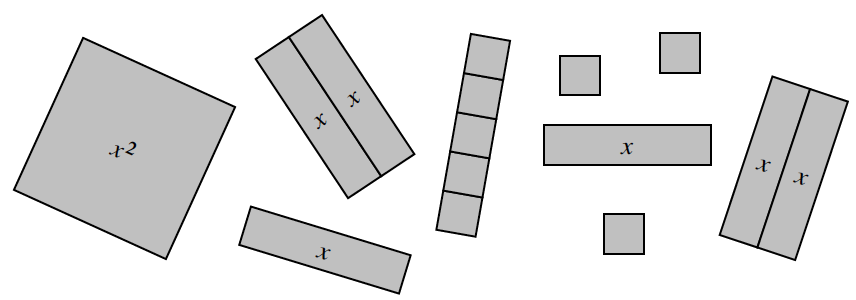• Use mathematical symbols (numbers, variables, and operations) to record the area of this collection of tiles.

• Write at least three different algebraic expressions that represent the area of this tile collection.

4-72.

Put the tiles pictured in each collection below on your table.  Then work with your team to find the area as you did in problem 4-71.

1. 4-72a Student eTool (CPM)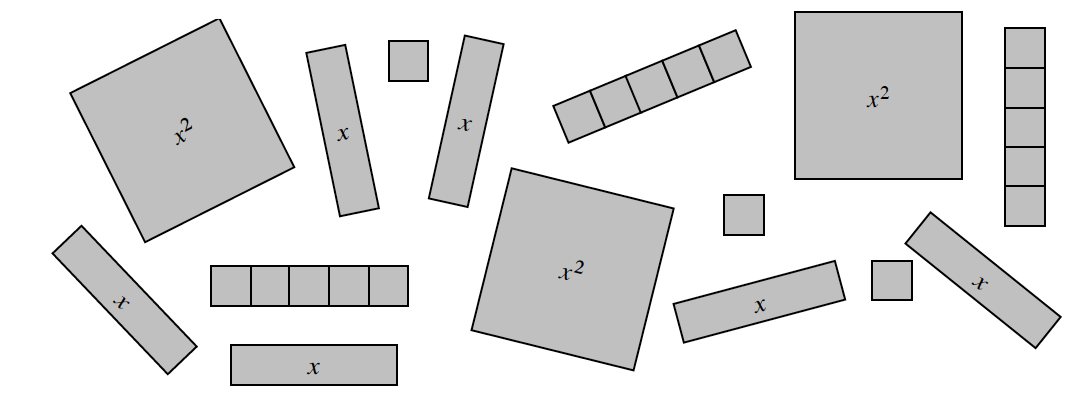1. 4-72b Student eTool (CPM)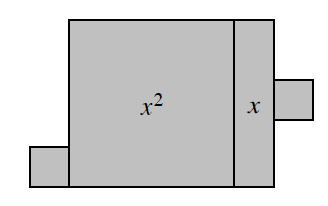1. 4-72c Student eTool(CPM)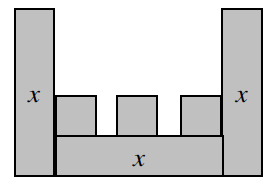4-73.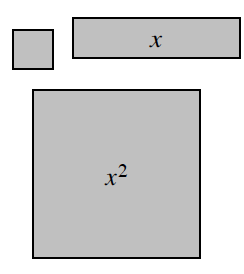The perimeter of each algebra tile can be also written as an expression using variables and numbers.

1. Write at least two different expressions for the perimeter of each tile shown at right

2. Which way of writing the perimeter seems clearest to you?  What information can you get from each expression?

1. Lianna wrote the perimeter of the collection of tiles below as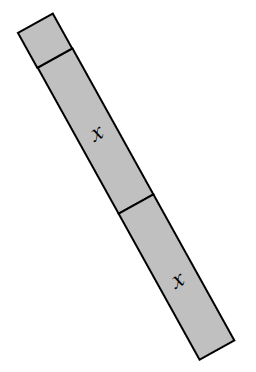2x+1+1+1+2x+1 units, but her teammate Jonah wrote it as 4x+4.  How are their expressions different?

1. Which expression represents the perimeter?

4-74.

The expressions that you have written to represent area and perimeter are made up of terms that are separated by addition and subtraction.

1.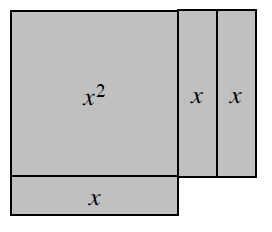Write an expression for the perimeter of the figure at right.

2. How many x lengths are represented in the expression in part (a)?  How many unit lengths?

3. Combining like terms (like terms contain the same variable raised to the same power) is a way of simplifying an expression.  Rewriting the perimeter of the shape above as P=4x+6 combines the separate xterms as 4x and combines the units in the term 6.

Assignment: 4.3.1 Homework

Handouts

Intro to Algebra Tiles & Expressions

Intro to Algebra Tiles & Expressions #24-85.

Using algebra tiles on your desk or the 4-85 Student eTool (CPM), make the shapes shown below.  Trace each shape and label the length of each side on your drawing.  With your team, find and record the total perimeter and area for each shape.  If possible, write the perimeter in more than one way.

1.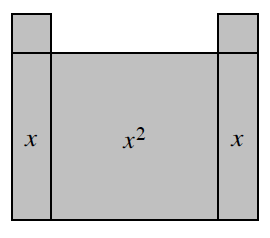2.3.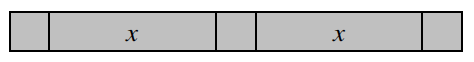4-86.

In problem 4-85, x is a variable that represents a number of units of length.  The value of x determines the size of the perimeter and area of the shape.

Using the shapes from problem 4-85, sketch and label each shape with the new lengths given below.  Then evaluate each expression for the given value of the variable.  That is, rewrite the expressions, replacing the variable with the number given, and then simplify them to determine the perimeter and area of each shape.

1. x=6 for all three shapes

2. x=12 for all three shapes

3. Compare your method for finding perimeter and area with the method your teammates used.  Is your method the same as your teammates’ methods?  If so, is there a different way to find the perimeter and area?  Explain the different methods.

4-87.

Build each of the shapes below using algebra tiles or explore using 4-87 Student eTool (CPM).  Look carefully at the lengths of the right sides.

1.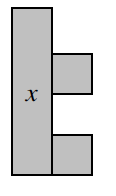1.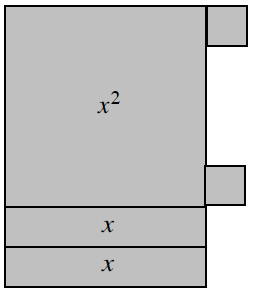1. Discuss with your team how to label the length of the right side of each figure.  Label each length on your paper.  Explain your reasoning.

2. Find the perimeter of each figure.  Write the perimeter in simplest form by combining the like terms.

4-88. Build the shape at right using algebra tiles.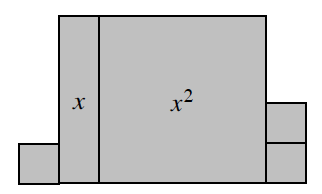1. x=5 units

1. x=3 units

1. x=2 units

1. x=1 unit

4-90.

Build the following expressions with algebra tiles or use the 4-90 Student eTool (CPM).  Then rewrite the expression a different way.  Remember that parentheses in an algebraic expression allow you to show that tiles are grouped together.

1. 4(2x+3)

2. 12x+18

3. 10+15x (Hint: Divide into as many equally-sized groups as possible)

4-91.

You have been writing expressions in different ways to mean the same thing.  These expressions depend on whether you see tiles grouped (like four sets of 2x+3 in part (a) of problem 4-90) or whether you see separate tiles (eight x-tiles and 12 unit tiles).  These two expressions are equivalent based on a mathematical property called the Distributive Property.

Use the Distributive Property to write an equivalent expression for 21x+7.  See if you can do it by visualizing tiles.

Assignment: 4.3.2 Homework